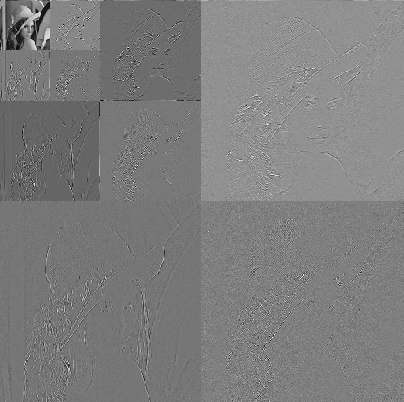# Multiscale analysis

The objective of multiscale analysis is to study signal (time-scale) or image (scale-space) singularities at different scales or resolutions .

A powerful tool is the Wavelet Transform that works as derivative operator at different scales . It provides a compact representation of the original signal and allows to also exploit inter and intra scale redundancy of its coefficients. It is well-known its persinstency property: "large/small values of wavelet coefficients tend to propagate across scales" . It can be easily seen in the following images where there are the original image (Lena) and its wavelet coefficients.An interesting problem is to link wavelet coefficients at a given (intra) scale and/or along (inter) scales, since many signal processing problems (compression, denoising, retrieval etc.) strongly depend on it.

1. A. Rosenfeld, M. Thurston, Edge and curve detection for visual scene analysis, IEEE Transactions on Comput., Vol. C-20, pp. 562-569, May 1971.

2. S. Mallat, A Wavelet Tour of Signal Processing, Academic Press, 1998.

3. M. S. Crouse, R. D. Nowak, R. G. Baraniuk, Wavelet-based Statistical Signal Processing using Hidden Markov Models, IEEE Transactions on Signal Processing, Vol. 46, No. 4, pp. 886-902, April 1998.